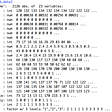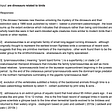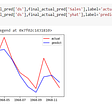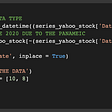# Preprocessing time series to windowed datasets

A time-series problem relies on using past inputs to determine future timesteps. This may work if the lagged time events are correlated with the present. Many models may solve this problem; however, I will only talk about data preparation. I tripped over while working with time series because I didn’t understand some of the following preprocessing concepts.

1. Windowing
2. Single Output vs. Multiple Output
3. Single Timestep vs. Multiple Timestep

Windowing

To window means to take a dataset and partition it into subsections (which increases the dimension shape of the dataset). In traditional machine learning, more input data tends to be better. However, in time series, it might not be the case.

For example, let’s say I have a dataset of 100 rows (x 1 column) and want to use the previous input(t-1) to determine t. The dataset can be sliced from the shape (100, 1) to X (99, 1, 1) and y (99, 1, 1). The one matrix (of 100row x 1column) is transformed into 2 tensors: 99 matrices each of 1 row and 1 column where the row is the number of time and column is the number of features.

Please note, I have lost one row because I can not include t=0 into y as I have missing X values at t = -1(minus). The input values can be increased to any length(as long as not greater than the dataset’s size). For example, I now want to input the previous 10 rows as inputs to determine the next time step(X = [t-5, t-4, t-3, t-2, t-1], y = [t]). This will result in X (90, 10, 1) and y (90, 1, 1).

For the previous example, there was only one variable to determine the next step. However, if the original dataset has more than one variable (for example, 5 features), then the transformation should result from the original dataset (100, 5) to X (90, 10, 5) and y (90, 1, 1). The X values are 90 matrices with 10 rows and 2 columns per matrix.

Single Output vs. Multiple Output

It might also be possible to have more than one y target for a single timestamp. With the previous example, if the output was the next timestamp value for all 5 features, then the original dataset (100, 5) will be transformed to X (90, 10, 5) and y (90, 1, 5).

One way to treat this problem is to have multiple models or weights, one for each target variable.

A few regression models can output multiple targets seamlessly, such as Linear Regression, Decision Tree Regressor, and Neural Networks. However, some machines such as SVR might need some manipulation to output multiple targets.

Single Timestep vs Multiple Timestep

Up until now, I have only discussed setting up the data to predict one timestamp. It might be of interest to be able to predict many time intervals.

Recap of the preprocessing so far:

1. Original dataset 100 rows x 5 features
2. The windowed input is 10 rows.
3. The windowed output is 1 row.
4. 5 input features
5. 5 output features
6. X.shape (90, 10, 5) ; y.shape (90, 1, 5)

In addition to t+1, I would also like to predict t+2. The windowed dataset should change from original (100, 5) to X (89, 10, 5) and y(89, 2, 5). Please note that I have lost some data due to the lack of endpoints at t = 101. Therefore, the X’s last matrix should stop at index number 97(in zero start index format), where values for index number 98 and 99 are the values for t+1 and t+2, respectively.

Single Shot

One approach to predicting multiple times is to use the input variables to predict t+1 and t+2 independently (This approach is similar to multiple outputs on a single timestamp, as stated before). This assumes there to be no correlation between t+1 and t+2, which may not be exactly what you want. Nonetheless, this approach still produces some promising results.

Autoregressive

Another approach to predicting multiple times is to predict one timestep and use the predicted value as an input (and dropping the oldest occurrence) to predict t+2.

It’s hard to say one approach would perform better than the other, and it’s advisable to try both techniques.

--

--

--

## More from Albert Um

Hello! My name is Albert Um.

Love podcasts or audiobooks? Learn on the go with our new app.

## Understanding Softmax Regression with an example in R — All About Analytics## Real or Not Real : With help of NLP lets work on twitter text when an accident is mentioned.## Semantic search using BERT embeddings## Vision Transformers: A Review — Part II## How to Access Stocks Market Data for Machine Learning on Python## Learning How To Classify Images Using Keras Part 2## Why “Linear Algebra” is cool ??## Albert Um

Hello! My name is Albert Um.

## Forecasting Hourly Electricity Consumption with ARIMAX, SARIMAX, and LSTM (Part I)## Univariate Time Series Forecasting using FBProphet## Time series with statsmodels: basic concepts needed for forecasting (2)## An easy start into Time-series Forecasting: A practical example using Darts library